• 本人近日将普通的串行选择排序算法改成了并行算法，在多核处理器上经测试速度大约是普通串行选择排序算法的1.5倍到2倍。网上并行选择排序算法相关的介绍基本没有看到，因此我将其发上来供大家参考，代码如下 ...
本人近日将普通的串行选择排序算法改成了并行算法，在多核处理器上经测试速度大约是普通串行选择排序算法的1.5倍到2倍。网上并行选择排序算法相关的介绍基本没有看到，因此我将其发上来供大家参考，代码如下

import java.math.BigInteger;

import static java.util.concurrent.locks.LockSupport.park;
import static java.util.concurrent.locks.LockSupport.unpark;

class ComValues
{
private BigInteger arr[];
private int nElem;

{
this.arr = arr;
this.nElem = num;
}

public BigInteger[] getArr ()
{
return arr;
}

public void setArr (BigInteger[] arr)
{
this.arr = arr;
}

public int getnElem ()
{
return nElem;
}

public void setnElem (int nElem)
{
this.nElem = nElem;
}

{
}

{
}

}

{
private ComValues cvs;
int max, left;
int out, in;

{
this.cvs = cvs;
thrd.start();
}

// This is the entry point for thread.
public void run ()
{
int nElems = cvs.getnElem();
BigInteger[] a = cvs.getArr();

try
{
left = 0;
out = nElems - 1;
do
{
max = out; // maxima
for (in = out - 1; in >= left; in--) // inner loop
if (a[in].compareTo(a[max]) > 0) // if max smaller,
max = in; // we have a new max
left++;
park();

out--;
} while (out >= (nElems % 2 == 0 ? nElems / 2 : nElems / 2 + 1)); // outer loop
}
catch (Exception e)
{
e.printStackTrace();
}
}
}

class ArraySel
{
private BigInteger[] a; // ref to array a
private int nElems; // number of data items
// --------------------------------------------------------------

public ArraySel (int max) // constructor
{
a = new BigInteger[max]; // create the array
nElems = 0; // no items yet
}

// --------------------------------------------------------------
public void insert (BigInteger value) // put element into array
{
a[nElems] = value; // insert it
nElems++; // increment size
}

// --------------------------------------------------------------
public void display () // displays array contents
{
for (int j = 0; j < nElems; j++) // for each element,
System.out.print(a[j].longValue() + " "); // display it
System.out.println("");
}

// --------------------------------------------------------------
public void selectionSort ()
{
int out, in, min, max, outTh;
int right = nElems - 1;

for (out = 0; out <= nElems / 2 - 1; out++) // outer loop
{
min = out; // minimum
for (in = out + 1; in <= right; in++) // inner loop
if (a[in].compareTo(a[min]) < 0) // if min greater,
min = in; // we have a new min

park();
max = SSRT.max;
outTh = SSRT.out;

swap(out, min);
if (!(max == out && outTh == min))
{
if (max == out)
swap(outTh, min);
else
swap(outTh, max);
}
unpark(SSRT.thrd);
right--;
} // end for(out)
//        service.shutdown();
} // end selectionSort()
// --------------------------------------------------------------

private void swap (int one, int two)
{
BigInteger temp = a[one];
a[one] = a[two];
a[two] = temp;
}
// --------------------------------------------------------------
} // end class ArraySel

class SelectSortApp
{
public static void main (String[] args)
{
int maxSize = 5000; // array size
ArraySel arr; // reference to array
arr = new ArraySel(maxSize); // create the array

for (int index = 0; index < maxSize; index++)
{
long n = (long) (java.lang.Math.random() * (maxSize - 1));
arr.insert(new BigInteger(Long.toString(n)));               // insert items
}

arr.display(); // display items

arr.selectionSort(); // selection-sort them

arr.display(); // display them again
} // end main()
} // end class SelectSortApp



展开全文java
• 本章将介绍一些常用的排序算法，有常用的串行排序，如冒泡排序选择排序、插入排序、奇偶排序；还有对奇偶排序并行实现方法。一、串行排序直接上代码public static void main(String[] args) { int[] array = {9...
本章将介绍一些常用的排序算法，有常用的串行排序，如冒泡排序、选择排序、插入排序、奇偶排序；还有对奇偶排序的并行实现方法。一、串行排序直接上代码public class Sorts {		public static void main(String[] args) {		int[] array = {9,8,23,34,65,78,3,46,24};		//		sortBubble(array); //冒泡		//		selectSorts(array); //选择排序		//		insertSorts(array);  //插入排序				oddEvenSorts(array); //奇偶排序				for (int i = 0; i < array.length; i++) {			System.out.print(array[i]+"-");		}	}		/**	 * 冒泡排序	 * 2018年6月15日	 * @param array	 */	private static void sortBubble(int[] array) {		for (int i = 0; i < array.length; i++) {			for (int j = i+1; j < array.length; j++) {				if (array[i] > array[j]) {					int temp = array[i];					array[i] = array[j];					array[j] = temp;				}			}		}	}		/**	 * 选择排序	 * 2018年6月15日	 * @param array	 */	private static void selectSorts(int[] array) {		for (int i = 0; i < array.length; i++) {			int min = i;			for (int j = i+1; j < array.length; j++) {				if (array[min] > array[j]) {					min = j;				}			}						if (array[i] > array[min]) {				int temp = array[i];				array[i] = array[min];				array[min] = temp;			}		}	}		/**	 * 插入排序	 * 2018年6月19日	 * @param array	 */	private static void insertSorts(int[] array) {		for (int i = 1; i < array.length; i++) {			int temp = array[i];			int j ;			for (j = i-1; j>=0 && temp < array[j]; j--) {					array[j+1] = array[j];			}			array[j+1] = temp;		}	}		/**	 * 奇偶排序	 * 2018年6月27日	 * @param array	 */	private static void oddEvenSorts(int[] array){		int flag = 1,start = 0;		while (flag == 1 || start == 1) {			flag = 0;			for (int i = start; i < array.length-1; i+=2) {				if (array[i] > array[i+1]) {					int temp = array[i];					array[i] = array[i+1];					array[i+1] = temp;					flag = 1;				}			}			if (start == 0) 				start = 1;			else 				start = 0;		}	}}二、并行排序奇偶排序的并行实现方法，在串行奇偶排序的基础上进行改造。/** * 并行 奇偶排序 * 2018年6月27日 */public class OddEvenSorts {	static int[] array = {9,8,23,34,65,78,3,46,24};	private static int flag = 1;	public static synchronized int getFlag() {		return flag;	}	public static synchronized void setFlag(int flag) {		OddEvenSorts.flag = flag;	}	static class OddEvenTasks implements Runnable{		int i;		CountDownLatch latch ;		public OddEvenTasks(int i, CountDownLatch latch) {			this.i = i;			this.latch = latch;		}		@Override		public void run() {			if (array[i] > array[i+1]) {				int temp = array[i];				array[i] = array[i+1];				array[i+1] = temp;				setFlag(1);			}			latch.countDown();		}	}		public static void oddEvenSort(int[] array) throws InterruptedException{		int start = 0;		ExecutorService executorService = Executors.newCachedThreadPool(); //创建线程池		while (getFlag() == 1 || start == 1) {			setFlag(0);			CountDownLatch latch = new CountDownLatch(array.length/2-(array.length%2==0?start:0));			for (int i = start; i < array.length-1; i+=2) {				executorService.submit(new OddEvenTasks(i, latch));			}			latch.await();			if (start == 0) 				start = 1;			else 				start = 0;		}	}	public static void main(String[] args) throws InterruptedException  {		oddEvenSort(array);		for (int arr : array) {			System.out.print(arr+"-");		}	}}
展开全文排序算法 并行奇偶排序 插入排序
• ## 并行排序

千次阅读 2019-04-20 14:05:34
并行排序算法是计算机并行计算能力大大发展之后，为了提高排序效率而提出的算法。 原有的的排序算法都是给定了数据再进行排序，排序的效率很大程度上取决于数据的好坏，例如快速排序、归并排序。并行排序则是一类...
个人博客网址：http://hyperparameter.cn/
并行排序算法是计算机并行计算能力大大发展之后，为了提高排序效率而提出的算法。
原有的的排序算法都是给定了数据再进行排序，排序的效率很大程度上取决于数据的好坏，例如快速排序、归并排序。并行排序则是一类完全不同的排序方法，它的所有比较操作都与数据无关。
并行排序的表示方式是排序网络，其主要构件就是Batcher比较器，通过组合比较器构建网络来完成排序功能。Batcher比较器指在两个输入端给定输入x,y，再在两个输出端输出最大值$\text{max}\{x,y\}$和最小值$\text{min}\{x,y\}$。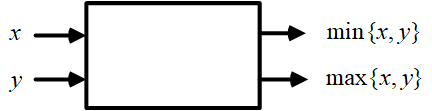传统排序算法网络
实际上，传统的某些排序算法（无条件比较的排序算法1）也可以用网络的形式表示，例如归并排序和快速排序就无法通过比较器构成的电路网络表示，因为无法预先知道后面的条件交换操作会在什么位置进行，换种说法就是，比较器构成的电路网络中必须不考虑输入就确定结构。
以冒泡排序举例，其核心是依次得出序列中的最值，其网络结构如下图所示（经调整过的冒泡排序网络，因为第$n$轮冒泡和第$n-1$轮冒泡在相差两个时间步后不再相互依赖，因此有一定的流水线并行性）。但是在并行硬件的背景下，可以看出这种排序算法仍有很大的改善空间（网络中的空白很多）。这是由于这类排序依赖于邻近数据的相对大小关系，不能很好地利用并行计算的资源。因此最直接的改良思路就是打破排序过程中数据间的依赖性，由此得到了奇偶合并网络和双调排序网络。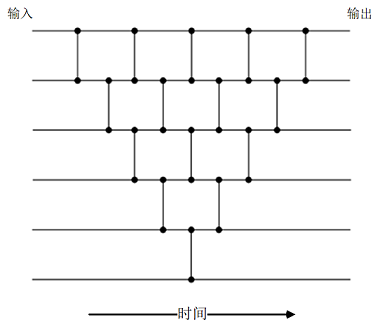冒泡排序网络
奇偶合并网络
首先考虑奇偶排序方法，该方法主要有三个步骤：

选取所有奇数列的元素与其右侧相邻的元素进行比较，将较小的元素排序在前面；
选取所有偶数列的元素与其右侧相邻的元素进行比较，将较小的元素排序在前面；
重复前面两步，直到所有序列有序为止。

这是最基础的奇偶排序算法，其网络表示如下，该方法的平均时间复杂度为$O\left(n \log n\right)$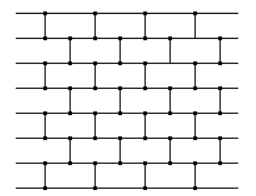奇偶排序算法2
奇偶合并网络就是结合了两个部分：归并排序得到两个有序的子序列，以及最后对两个子序列的合并过程。如下图所示，两个蓝色方框内依次完成归并排序的两层得到两个有序的子序列。最后的合并过程（后面一个红色框），则是首先对所有的奇数项和偶数项分别递归地合并，然后在排序后的第i个偶数项和第i+1个奇数项之间设立Batcher比较器分别进行最后的调整。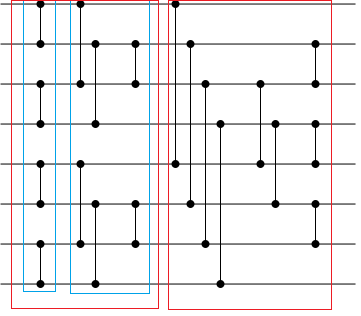平均和最差时间复杂度都是$O\left(\log ^{2}(n)\right)$，空间复杂度则是$O\left(n \log ^{2}(n)\right)$。奇偶归并网络的Python实现如下，
def oddeven_merge(lo, hi, r):
step = r * 2
if step < hi - lo:
yield from oddeven_merge(lo, hi, step)
yield from oddeven_merge(lo + r, hi, step)
yield from [(i, i + r) for i in range(lo + r, hi - r, step)]
else:
yield (lo, lo + r)

def oddeven_merge_sort_range(lo, hi):
""" sort the part of x with indices between lo and hi.

Note: endpoints (lo and hi) are included.
"""
if (hi - lo) >= 1:
# if there is more than one element, split the input
# down the middle and first sort the first and second
# half, followed by merging them.
mid = lo + ((hi - lo) // 2)
yield from oddeven_merge_sort_range(lo, mid)
yield from oddeven_merge_sort_range(mid + 1, hi)
yield from oddeven_merge(lo, hi, 1)

def oddeven_merge_sort(length):
""" "length" is the length of the list to be sorted.
Returns a list of pairs of indices starting with 0 """
yield from oddeven_merge_sort_range(0, length - 1)

def compare_and_swap(x, a, b):
if x[a] > x[b]:
x[a], x[b] = x[b], x[a]

双调排序网络
1968年，肯特州立大学的计算机教授Kenneth Batcher设计出一种基于比较单元的电路，可以用$0.5\log_2^n\cdot(\log_2^n+1)​$并行时间步完成长度为$n​$的序列排序，并命名为双调合并网络（bitonic merger）。其中，双调序列定义为一个先单调递增再单调递减（或者先单调递减再单调递增）的序列。即存在一个$0 \leq k \leq n-1​$，使得$<a_{0}, a_{1}, \dots, a_{k-1}> ​$为升序序列，$<a_{k}, a_{k+1}, \dots, a_{n-1}>​$为降序序列。
从以上定义可以推出双调序列具有的一个特点，即：如果$n​$为偶数，且$<a_{0}, a_{1}, \dots, a_{n / 2-1}>​$为升序序列，$<a_{0}, a_{1}, \dots, a_{n / 2-1}>​$为降序序列，则以下两个序列都是双调序列，
$S_{1}=<\min \left(a_{0}, a_{n / 2}\right), \min \left(a_{1}, a_{n / 2+1}\right), \dots, \min \left(a_{n / 2-1}, a_{n-1}\right)>​$
$S_{2}=<\max\left(a_{0}, a_{n / 2}\right), \max\left(a_{1}, a_{n / 2+1}\right), \dots, \max\left(a_{n / 2-1}, a_{n-1}\right)>$
借助以上推论可以得到双调归并排序的算法，示例如下图所示，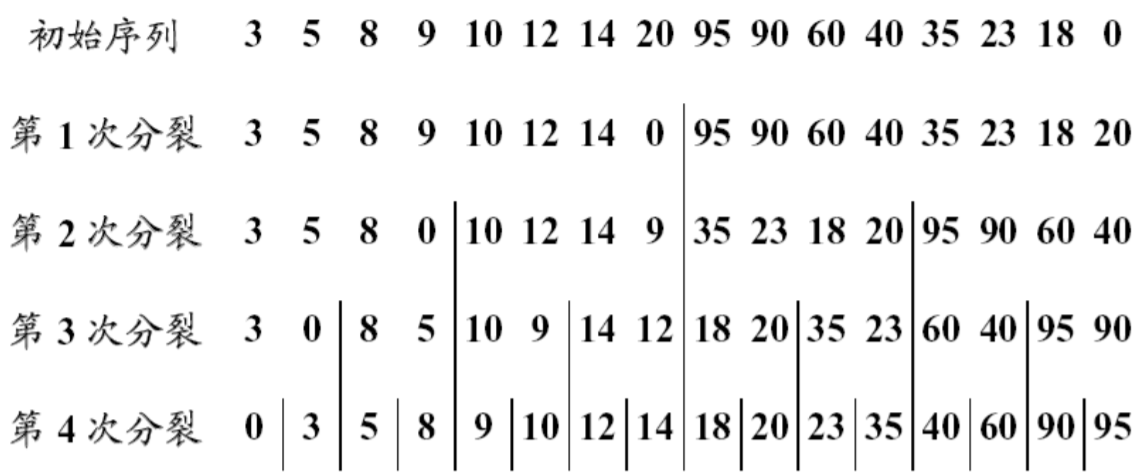上述归并排序算法的电路网络可以表示如下图（16路输入），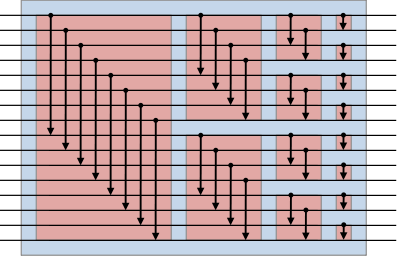那么现在就有一个问题是如何得到最初的双调序列（初始序列），这个部分就是通过下图的红框部分完成的，再拼接上双调归并排序的部分，可以看出整个双调排序网络都是基于Batcher比较器搭建而成。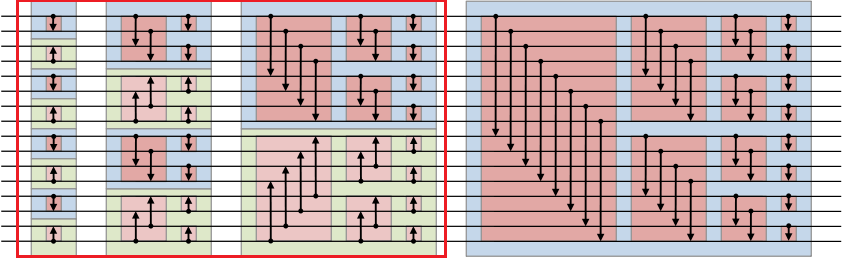双调排序网络分析3
下面这个是一个8输入的双调排序网络示例，可以看出也是由这两个部分组成。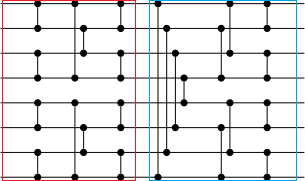8输入的双调排序网络示例
考虑双调排序网络的时间复杂度，因为该方法不依赖数据特性，最差时间复杂度和最好时间复杂度都是$O\left(\log ^{2}(n)\right)$，空间复杂度则是$O\left(n \log ^{2}(n)\right)$。
双调排序网络的Python实现如下，
def bitonic_sort(up, x):
if len(x) <= 1:
return x
else:
first = bitonic_sort(True, x[:len(x) // 2])
second = bitonic_sort(False, x[len(x) // 2:])
return bitonic_merge(up, first + second)

def bitonic_merge(up, x):
# assume input x is bitonic, and sorted list is returned
if len(x) == 1:
return x
else:
bitonic_compare(up, x)
first = bitonic_merge(up, x[:len(x) // 2])
second = bitonic_merge(up, x[len(x) // 2:])
return first + second

def bitonic_compare(up, x):
dist = len(x) // 2
for i in range(dist):
if (x[i] > x[i + dist]) == up:
x[i], x[i + dist] = x[i + dist], x[i] #swap

0-1原理证明
那么给定一个排序网络，如何确定它是真的能够正确地排序任意输入呢？**0-1原理（0-1 Principle）**是由计算机教授高德纳（Donald Ervin Knuth）提出来的，他在《计算机程序设计艺术》的第三卷5.3.4节：排序与选择中，提出并论证了这个原理。该原理叙述如下：
如果一个排序网络能够正确地对任何0-1序列排序，那么它就能对任意数组成的任意序列正确排序。
因此为了验证一个输入排序网络的正确性，我们不必检验所有数字构成的任意长为n的序列，而只需检验 $2^{n}$个0-1序列就足以验证排序网络是否能正确排序了。这篇文章通过数学归纳法证明了奇偶合并网络能够对任何0-1序列排序，也就证明了它就能对任意数组成的任意序列正确排序。1
非2次幂输入的排序网络
需要注意的是，以上两种提到的排序网络都假设了输入是2的整数次幂，保证了排序过程中的划分完整性。但是实际中，这是很难达到的目标，为了解决这个问题，最简单的方法就是填充，通过填充值并在排序结束后剔除以满足这个条件。还有一种方法就是对排序网络进行改进以能够解决任意$n​$的输入4
输入数据为6的排序网络设置如下，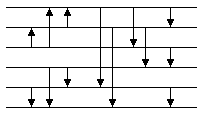该方法的实现如下，
public class BitonicSorterForArbitraryN implements Sorter
{
private int[] a;
private final static boolean ASCENDING=true;    // sorting direction

public void sort(int[] a)
{
this.a=a;
bitonicSort(0, a.length, ASCENDING);
}

private void bitonicSort(int lo, int n, boolean dir)
{
if (n>1)
{
int m=n/2;
bitonicSort(lo, m, !dir);
bitonicSort(lo+m, n-m, dir);
bitonicMerge(lo, n, dir);
}
}

private void bitonicMerge(int lo, int n, boolean dir)
{
if (n>1)
{
int m=greatestPowerOfTwoLessThan(n);
for (int i=lo; i<lo+n-m; i++)
compare(i, i+m, dir);
bitonicMerge(lo, m, dir);
bitonicMerge(lo+m, n-m, dir);
}
}

private void compare(int i, int j, boolean dir)
{
if (dir==(a[i]>a[j]))
exchange(i, j);
}

private void exchange(int i, int j)
{
int t=a[i];
a[i]=a[j];
a[j]=t;
}

// n>=2  and  n<=Integer.MAX_VALUE
private int greatestPowerOfTwoLessThan(int n)
{
int k=1;
while (k>0 && k<n)
k=k<<1;
return k>>>1;
}

}


OI之外的一些东西：简单谈谈排序网络 ↩︎ ↩︎

Batcher odd–even mergesort ↩︎

Bitonic sorter ↩︎

Bitonic sorting network for n not a power of 2 ↩︎


展开全文排序网络
• ## 并行快速排序

千次阅读 2014-04-09 16:10:45
并行快速排序 感谢网友浅水清流投递本稿。 并发算法是多核时代开始流行的技术趋势，比如tbb，ppl都提供了大量有用的并发算法。 经典算法中，排序是一个很适合采用分治法并发的场合，比如快速排序。 ...

并行快速排序

感谢网友浅水清流投递本稿。

并发算法是多核时代开始流行的技术趋势，比如tbb，ppl都提供了大量有用的并发算法。

经典算法中，排序是一个很适合采用分治法并发的场合，比如快速排序。

常规的快速排序，先在数组中选取一个基准点，将数组分区为小于基准点和大于基准点（相同的数可以到任一边），对分区的子数组递归的执行分区的操作，当子数组长度为1时退出递归。此时数组就完成了排序过程。

01

int partition(int*
array, int left, int right)

02

{

03

        int index
= left;

04

        int pivot
= array[index];

05

        swap(array[index],
array[right]);

06

        for (int i=left;
i<right; i++)

07

        {

08

                if (array[i]
> pivot)    //
降序

09

                        swap(array[index++],
array[i]);

10

        }

11

        swap(array[right],
array[index]);

12

        return index;

13

}

14

15

void qsort(int*
array, int left, int right)

16

{

17

        if (left
>= right)

18

                return;

19

        int index
= partition(array, left, right);

20

        qsort(array,
left, index - 1);

21

        qsort(array,
index + 1, right);

22

}

对快排的过程分析可以发现，分区以及对子数组排序的过程均可以并发执行，这里首先对数组进行分区，生成分区数组，为了保证不同分区不受到影响需要先完成分区再进行排序。

01

template <typename key, typename container
>void parallel_sort(container
& _container)template <typename key, typename container
>

02

void partition_less(std::vector<key>
* vless, container * _container, key privot){

03

for(size_t i
= 0; i < (*_container).size(); i++){

04

        if ((*_container)[i]
< privot){

05

            vless->push_back((*_container)[i]);

06

        }

07

    }

08

}

09

10

template <typename key,  typename container
>

11

void partition_more(std::vector<key>
* vmore, container * _container, key privot){

12

for(size_t i
= 0; i < (*_container).size(); i++){

13

        if ((*_container)[i]
>= privot){

14

            vmore->push_back((*_container)[i]);

15

        }

16

    }

17

}

在完成分区之后，递归执行排序操作，并将排序好的分区重新写入待排序数组。

01

template <typename key, typename container
>

02

int sort_less(container
* _container, std::vector<key> & vless, boost::atomic_uint32_t * depth){

03

    parallel_sort_impl<key>(&vless,
*depth);

04

05

    for(size_t i
= 0; i < vless.size(); i++){

06

        (*_container)[i]
= vless[i];

07

    }

08

09

    return 0;

10

}

11

12

template <typename key, typename container
>

13

int sort_more(container
* _container, std::vector<key> & vmore, boost::atomic_uint32_t * depth){

14

    parallel_sort_impl<key>(&vmore,
*depth);

15

16

    size_t pos
= (*_container).size()-vmore.size();

17

    for(size_t i
= 0; i < vmore.size(); i++){

18

        (*_container)[i+pos]
= vmore[i];

19

    }

20

21

    return 0;

22

}

23

24

template <typename key, typename container
>

25

void parallel_sort_impl(container
* _container, boost::atomic_uint32_t & depth){

26

    if (_container->size()
< threshold || depth.load() > processors_count()){

27

        std::sort(_container->begin(),
_container->end());

28

    }else{

29

        key
privot = (*_container)[_container->size()/2];

30

31

    std::vector<key>
vless, vmore;

32

    auto
partition_result = std::async(std::launch::async, partition_less<key, container>, &vless, _container, privot);

33

    partition_more(&vmore,
_container, privot);

34

    partition_result.get();

35

36

        auto
result = std::async(std::launch::async, sort_less<key, container>, _container, vless, &depth);

37

        sort_more(_container,
vmore, &depth);

38

        result.get();

39

    }

40

}

这里采取了一个有趣的策略，就是通过数组的大小，计算出排序好的元素在原数组中的位置（这样即使是并发的访问数组，但是因为不同的线程各自访问的自己的下标位置，所以仍然是线程安全的），然后将排序好的数组直接写入到原数组，完成整个排序。

这里的并发采用了c++11中的promise:http://imcc.blogbus.com/logs/174131661.html

（全文完）如果您喜欢此文请点赞，分享，评论。

转载自：并行快速排序


展开全文算法
• 文章目录一、归并排序回顾二、Java并行编程框架三、RecursiveAction详解四、测试和效率分析 一、归并排序回顾 归并排序，想必大家都不陌生，它是我们学习排序算法和分治法的极好例子。它是稳定排序，且有稳定的O...
• 从古至今的难题 　 　在IT届有一道百算不厌其烦的题，俗称排序。...最后，在头脑风暴下，LZ又有幸认识了一位新朋友，名叫并行归并排序。接下来，咱们就一一认识一下，并且在最后来一次“算林大会”吧。
• 2019独角兽企业重金招聘Python工程师标准>>> ...
• 基于openMP的并行计数排序算法 这是云计算的作业，实现对某个算法或程序的性能优化，以前没有接触过，所以使用了比较简单上手的openMP来实现。 代码如下 #include <stdio.h> #include <omp.h> #include ...openmp
• 对于排序算法相信大家都不陌生，大部分排序的程序都是串行的排序算法，比如冒泡排序，插入...在介绍并行排序之前，我们有必要介绍一下奇偶排序，为了将串行排序算法改为并行排序，使用奇偶排序可以更加方便的修改...算法 多线程
• 并行归并排序在程序开始时，会将n/comm_comm个键值分配给每个进程，程序结束时，所有的键值会按顺序存储在进程0中。为了做到这点，它使用了树形结构通信模式。当进程接收到另一个进程的键值时，它将该键值合并进自己...
• 因为项目需要对大量数据进行排序计算top k，开始了解并行计算框架，接触了spark，spark都是用scala写的，所以为了了解spark，恶补了一阵scala语言。 这是一种非常简练的函数式语言，最让我感觉兴趣的就是它天然支持...scala java spark
• 对于数组排序，一般使用Arrays.sort()方法串行排序，Java8新增方法Arrays.parallelSort()并行排序。 例子，使用串行&并行排序数组： private void test() { int[] arr = getNumbers(); long start = ...
• 基于多线程的并行快速排序算法实现 1. 快速算法(Quick Sort)介绍 快速排序(Quick Sort)是一种经典的排序算法，基于递归实现，由于其实现方式简单可靠、平均时间复杂度为O(nlogn) （最坏情况O(n^2)), 被广泛采用。...
• 有一些排序算法如归并排序、快速排序等可以分解为子问题的算法是可以使用多线程来加速排序的，之前做了个小实验，测试了下自己写的MergeSort::parallelSort、QuickSort::parallelSort以及Arrays::sort、Arrays::...java 算法
• 并行排序方法，是指采用并行计算的方法对一组数据进行排序，理论上是在类似内排序的环境下，采用多核并行的方法让时间降低，排序的复杂度最好的情况下能降低至O(n)左右。排序的实质排序的实质是什么？这是一个不是...数据 class
• 在第六讲中，本文以冒泡排序 Bubble Sort、归并排序 Merge Sort 和排序网络中的双调排序 Bitonic Sort 为例， 讲解如何从数据结构课上学的串行并行排序方法转换到并行排序，并附GPU实现代码。 在并行方法中，我们将...cuda
• 双调排序是data-independent的排序， 即比较顺序与数据无关的排序方法， 特别适合做并行计算，例如用GPU、fpga来计算。算法
• 因此多核环境中的排序一般需要用到并行排序算法。 并行排序算法和串行排序算法相比，会增加一些额外的开销，如计算开销或空间开销。那么在并行排序算法中，有那些需求呢？下面给出一些并行排序方面的需求供参考。...多核架构
• 用Parallel_For进行并行快速排序作者： Zhouweiming 周伟明 (32 篇文章) 日期： 五月 31, 2009 在 10:43 上午 用Parallel_For进行并行快速排序 注：本文主要内容摘自笔者所著的《多核计算与程序设计》一书，略有...parallel 任务调度 class 多线程
• 利用MPI实现快速排序并行算法，算法使用C语言实现
• 本文主要讨论并行排序算法的实现，将串行的奇偶排序算法并行化。同时本文也涉及MPI通信安全方面的讨论，MPI_SendRecv函数提供了有关进程通信的调度，用它替代send和recv函数使程序更安全算法 c++
• 双调排序是data-independent的排序， 即比较顺序与数据无关的排序方法， 特别适合做并行计算，例如用GPU、fpga来计算。 1、双调序列 在了解双调排序算法之前，我们先来看看什么是双调序列。  (1)先单调递增后......